Today: image co-ordinates, range, nested range, make drawing to figure out co-ords

## Coding Style 1.0 - Tactics

CS106A doe not just teach coding. It has always taught how to write clean code with good style. Your section leader will talk with you about the correctness of code, but also pointers for good style.

All the code we show you will follow PEP8, so just picking up the style tactic that way is the easiest.

Python Guide Style-1, we'll pick out a few things today (for hw1), re-visit it for the rest later.

• Today:
• indent 4 spaces after the colon (:), for def/while/if etc.
See all our examples
• 1 space between elements
e.g. `1 + 2 * 3`
• We prefer single quote mark for strings like `'red'`
• The word `pass` in Python means is a placeholder that does nothing
Often `pass` in the code marks the place where you add code
Remove the `pass` when you put your code in, it's just a placeholder

## Strategy Note: Detail Oriented

• "Detail Oriented" - frequently seen on the resume!
• Book: Thinking Fast and Slow, by Kahneman
• Mostly your brain is not paying much attention and that's fine
• Get to Aqua-Stripe problem below...
Slow down and work the details
Make a rough drawing to plot how the details fit together
Then write the key line of code

## Recall: `image.get_pixel(x, y)`

• An image function that accesses one pixel
• `image.get_pixel(x, y)`
• Returns a reference to the pixel at x,y
• Store reference in a variable, use .red .green on it
• Typically we use "pixel" as the variable name for this
```# For the pixel at x=4 y=2 in "image",
# set its red value to 0
pixel = image.get_pixel(4, 2)
pixel.red = 0
```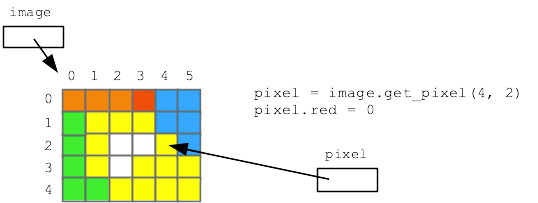## Recall: range(n)

• We have the range(n) function
• Returns a series of numbers 0..n-1
e.g. `range(10)` -> 0, 1, 2, 3 .. 9
• With image width and height
range(n) is perfect for generating x and y values into an image

## Example: Darker-Nested

Here is a version of our earlier "darker" algorithm, but written using nested range() loops. The nested loops load every pixel in the image and change the pixel to be darker. On the last line `return image` outputs the image at the end of the function (more on "return" next week). Run it to see what it outputs. Then we'll look at the code in detail.

```def darker(filename):
image = SimpleImage(filename)
for y in range(image.height):
for x in range(image.width):
pixel = image.get_pixel(x, y)
pixel.red *= 0.5
pixel.green *= 0.5
pixel.blue *= 0.5
return image
```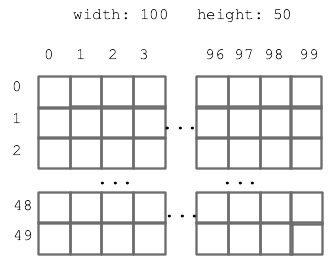## 1. `for y in range(image.height):`

Say the image height is 50. Then range(50) is the series 0, 1, 2 .. 49. Which is all the y values for this image, from top to bottom. The loop runs the y variable through 0, 1, 2 ... 49, one number for each iteration.

## 2. `for x in range(image.width):`

Say the image width is 100. The range(100) is the series 0, 1, 2 .. 99 which are the x index numbers from left to right. The loop runs the variable x through 0, 1, .. 99, one number for each iteration.

## 3. Loop Nesting

• The for-y/for-x loops are nested
y loop = outer loop
x loop is nested (indented) inside, = inner loop
• Nested loop sequence:
• 1. Outer loop does first iteration, e.g. y = 0
• 2. Inner loop does all its iterations, x = 0, 1, 2, 3 ...
This gives us all y=0 coords: (0, 0), (1, 0), (2, 0), .. (99, 0)
• 3. Outer loop does second iteration, y = 1
• 4. Inner loop does all iterations again: x = 0, 1, 2, 3 ...
This gives us all y=1 coords: (0, 1), (1, 1), (2, 1), .. (99, 1)
• The effect is going through the rows top to bottom. Each row going left to right - like reading English text
• Demo: add in inner loop: `print('x:', x, 'y:', y)`
print() like this is a debugging trick we'll build on later
See a line printed for each pixel, showing the whole x,y sequence
Only do this with small images like we have here
Otherwise it's too much output
• Demo: try making x too limited, `range(image.width - 20)`
• Demo: what if we swap the roles of the y/x loops?
It works fine, just a different order of the pixels
Goes through the columns left-right. For each column, top to bottom

Here is a picture, showing the order the nested y/x loops go through all the pixels - all of the top y=0 row, then the next y=1 row, and so on.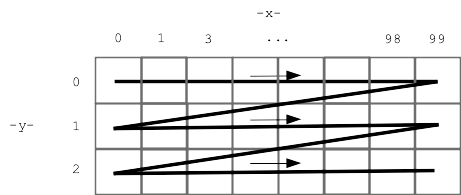## Nested y/x - Idiomatic

The nested y/x loop code has a lot of detail in it. The good news is, writing the loops this way to hit every pixel in an image is idiomatic. It's the same every time, so you can use it as a unit while you get used to its details.

## How to Make 2 Pixels Look The Same?

• Suppose variables a and b refer to two pixels in an image
• Make pixel b look the same as a .. how?
• Pixels look the same if they have the same RGB numbers
• Make b look the same as a with three = for red/green/blue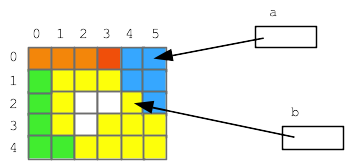```# Make pixel b look the same as pixel a
b.red = a.red
b.green = a.green
b.blue = a.blue
```

## How to Create a New, Blank Image?

Thus far the code has changed the original image. Now we'll create a new blank white "out" image and write changes to that.

```image = SimpleImage(filename)

# 1. create a blank white 100 x 50 image, store in variable named "out"
out = SimpleImage.blank(100, 50)

# 2. More realistic, create an out image the same size
# as the original
out = SimpleImage.blank(image.width, image.height)
```

## Code With Two Images - getPixel()

In the code below, we have two images "image" and "out" - can get a pixel in either image how? The key is which image is before the dot when the code calls get_pixel().This is the essence of noun.verb function call form. Which image do we address the get_pixel() function call to?

```# a points to pixel (10, 0) in original image
a = image.get_pixel(10, 0)

# b points to pixel (10, 0) in out image
b = out.get_pixel(10, 0)
```

## Example: Darker Out

Establish the basics on this one, do something interesting on the next one.

Darker algorithm, but creating a separate out image.

```def darker(filename):
image = SimpleImage(filename)
# Create out image, same size as original
out =  SimpleImage.blank(image.width, image.height)
for y in range(image.height):
for x in range(image.width):
pixel = image.get_pixel(x, y)
pixel_out = out.get_pixel(x, y)
pixel_out.red = pixel.red * 0.5
pixel_out.green = pixel.green * 0.5
pixel_out.blue = pixel.blue * 0.5
return out
```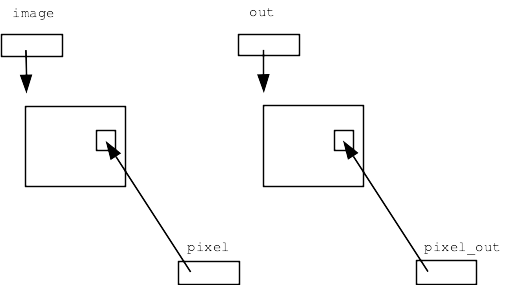• Standard nested loops go through all x,y
• "pixel" points into original image
• "pixel_out" points into out image
• Copy over the data
• Return "out" when done

## Exercise - Aqua Stripe

For the Aqua Stripe problem, produce an image with a 10 pixel wide aqua stripe on the left, with a copy of the original image next to it, like this: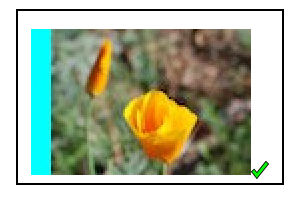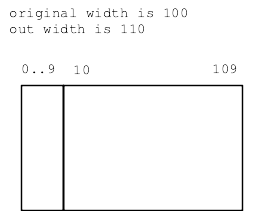## 1. Make out image - 10 pixels wider than original

```out = SimpleImage.blank(image.width + 10, image.height)
```

## 2. Produce the aqua stripe

• What are the x,y coordinates for the stripe?
What nested loops will hit them all?
• The blank image is white
• How to make aqua?
White is 255 255 255
Set red to 0
That leaves blue and green at 255 = aqua!

## 3. Loop To Copy Over Original - Drawing!

How to copy the data from the original to the right side of the output? Need to think about the x,y values, which are not the same in the two images.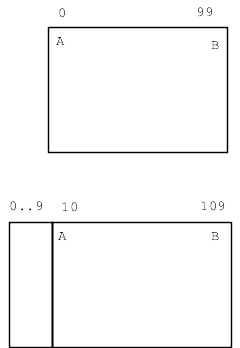For x,y in original, what is the corresponding x,y in out?

Make a little chart (here "x" means in the original image)

```pt   x     x_out
A    0     10
B    99    109

What's the pattern?
x_out = x + 10
```

Now we can write the key get_pixel() line below, to figure pixel_out for each original pixel.

## Aqua Stripe Solution

```def aqua_stripe(filename):
"""
Create an out image 10 pixels wider than the original.
(1) Set an aqua colored vertical stripe 10 pixels wide
at the left by setting red to 0.
(2) Copy the original image just to the right
of the aqua stripe. Return the computed out image.
"""
image = SimpleImage(filename)
# Create out image, 10 pixels wider than original
out = SimpleImage.blank(image.width + 10, image.height)
# Create the 10-pixel aqua stripe
for y in range(image.height):
for x in range(10):
pixel_out = out.get_pixel(x, y)
pixel_out.red = 0
# Copy the original over - make drawing to guide code here
for y in range(image.height):
for x in range(image.width):
pixel = image.get_pixel(x, y)
pixel_out = out.get_pixel(x + 10, y)  # the key line
pixel_out.red = pixel.red
pixel_out.green = pixel.green
pixel_out.blue = pixel.blue
return out
```

Experiments: try +11 instead of +10 - get bad-coord exception, Try +9, and image shifted slightly to left, can try the diff-stripes slider.

## Strategy

It's hard to write that get_pixel() line just doing it in your head. We make a drawing to get the details exactly right.

## Concrete Numbers

Notice that our drawing was not general - just picking width = 100 as a concrete example. A single concrete example was good enough to get our thoughts organized, and then the formula worked out actually was general.

## Off By One, OBO

A common form of error in these complex indexing algorithms is being "off by one", like using x = 100 when x = 99 is correct.

## Example - Mirror1

• This one is relatively simple, code is provided
• This problem will have two images in it
• Can call get_pixel() to obtain pixels in both
• Create a blank "out" image, twice as wide as original image:
`out = SimpleImage.blank(image.width * 2, image.height)`
• This problem:
• 1. Create "out" twice as wide as original
• 2. Copy original image to left half
• Look at the get_pixel() calls
• "pixel" is in original image
• "pixel_left" is in out image (left half)
• In this case the x,y coords are the same in both images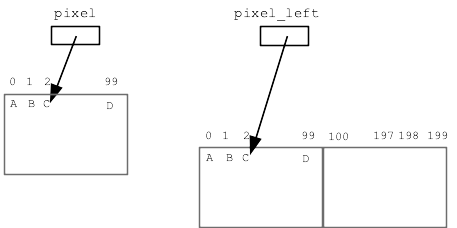```def mirror1(filename):
image = SimpleImage(filename)
# SimpleImage.blank(width, height) - returns a new
# image with size given in its 2 parameters.
# Here, create out image with width * 2 or first image:
out = SimpleImage.blank(image.width * 2, image.height)
for y in range(image.height):
for x in range(image.width):
pixel = image.get_pixel(x, y)
# left copy
pixel_left = out.get_pixel(x, y)
pixel_left.red = pixel.red
pixel_left.green = pixel.green
pixel_left.blue = pixel.blue
# right copy
# nothing!
return out
```

## Exercise - Mirror2

This a favorite example, bringing it all together. This algorithm pushes you to work out details carefully with a drawing, and the output is just neat.

Mirror2: Like mirror1, but also copy the original image to the right half of "out", but as a horizontally flipped mirror image. So the left half is a regular copy, and the right half is a mirror image. (Starter code does the left half).

## Mirror2 Strategy

I think a reasonable reaction to reading that problem statement is: uh, what? How the heck is that going to work? But proceeding carefully we can get the details right. Don't do it in your head.

Make a drawing of the image coordinates with concrete numbers, work out what the x,y coordinates are for input and output pixels. We'll go though the whole sequence right here.

• Like mirror1, place left-right swapped mirror image on right half
• A complex problem with coordinates, make a drawing
• Choose concrete numbers to work out an example
• e.g. say original width = 100
• Make diagram, say 4 ABCD "source" points
• Choose good variable names
Use var names to keep the roles clear in the algo
`pixel` - in source image, at x,y
`pixel_left` - left side of out
`pixel_right` - right side of out
• Key question:
For each x in the original image, what is the x for pixel_right in the out image?
```Here are 4 points in the original:
A: (0, 0)
B: (1, 0)
C: (2, 0)
D: (99, 0)

Sketch out where these points should land in the output.
What is x value for pixel_right for each of these?
```

## Sketch out ABCD Values

Try completing drawing with ABCD values. This is a great example of slowing down, working out the details. We start knowing what we want the output to look like, proceed down to the coordinate details.• Figure out the formula to compute out pixel_right x,y from source x,y
• (Demo: go through points, figure out formula)
• (Demo: put in wrong formula, see bad x,y error message)
• Strategy: concrete numbers + drawing .. work out code details
• Diff slider draws yellow bars where the output seems wrong to the systemABCD Table Solution

## mirror2 Solution Code

```def mirror2(filename):
image = SimpleImage(filename)
out = SimpleImage.blank(image.width * 2, image.height)
for y in range(image.height):
for x in range(image.width):
pixel = image.get_pixel(x, y)
# left copy
pixel_left = out.get_pixel(x, y)
pixel_left.red = pixel.red
pixel_left.green = pixel.green
pixel_left.blue = pixel.blue
# right copy
# this is the key spot
# have: pixel at x,y in image
# want: pixel_right at ??? to write to
pixel_right = out.get_pixel(out.width - 1 - x, y)
pixel_right.red = pixel.red
pixel_right.green = pixel.green
pixel_right.blue = pixel.blue
return out
```

## Debugging: Put in a bug

Remove the "- 1" from formula above, so out_x value is one too big. A very common form of Off By One error. What happens when we run it?

## Debugging Rule #1 - Read The Exception Message

An exception is an error cause by a line of code that halts the program at that point. The exception will have a message describing the low-level error, like a bad coordinate, and it will give a line number.

Just with those two facts - look at your code. Why is the code doing that? Sometimes just being pointed at the right line is enough for you to spot the bug.